### COS Function – Calc Cosine of Angle – Excel, VBA, G Sheets

This tutorial demonstrates how to use the Excel COS Function in Excel to calculate the cosine.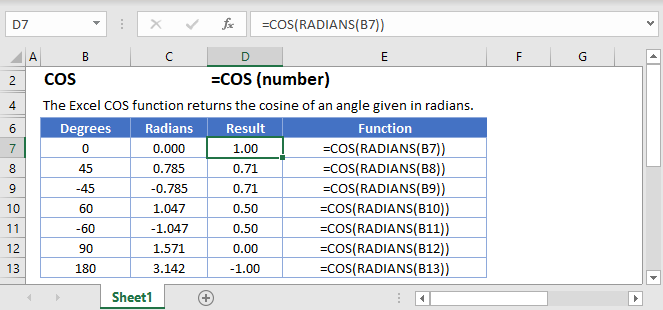## COS Function Overview

The COS function calculates the cosine of an angle in radians.

To use the COS Excel Worksheet Function, select a cell and type: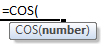(Notice how the formula inputs appear)

### COS Function Syntax and Inputs:

`=COS(number)`

number – A number that represents an angle in radians. This can be any real number from negative infinity to positive infinity.

AutoMacro - VBA Code Generator

## Creating a cosine curve

To create a cosine curve in Excel, we need to first choose our start and end points and then list out a lot of numbers. Let’s go from -2π up to 2π in increments of 0.1.

`=COS(C3)`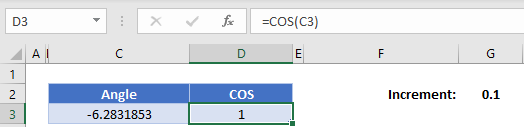Next, we’re going to add the 0.1 onto the angle and then calculate the cosine of that angle. Use the formula

`=C3+\$G\$2`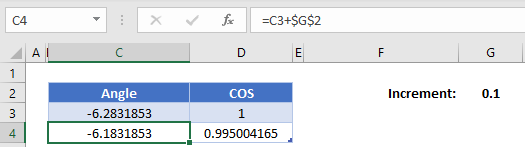(the \$ signs lock G2 so the formula will always reference that 0.1 even if we copy the formula!)

Now highlight both the new angle and cosine function that we’ve calculated, hold the handle, and drag it down until our angle reaches about 2π (about 6.28)

Highlight the entire range of both angles and cosines, click insert, find the graphs and select “Scatter with Smooth Lines”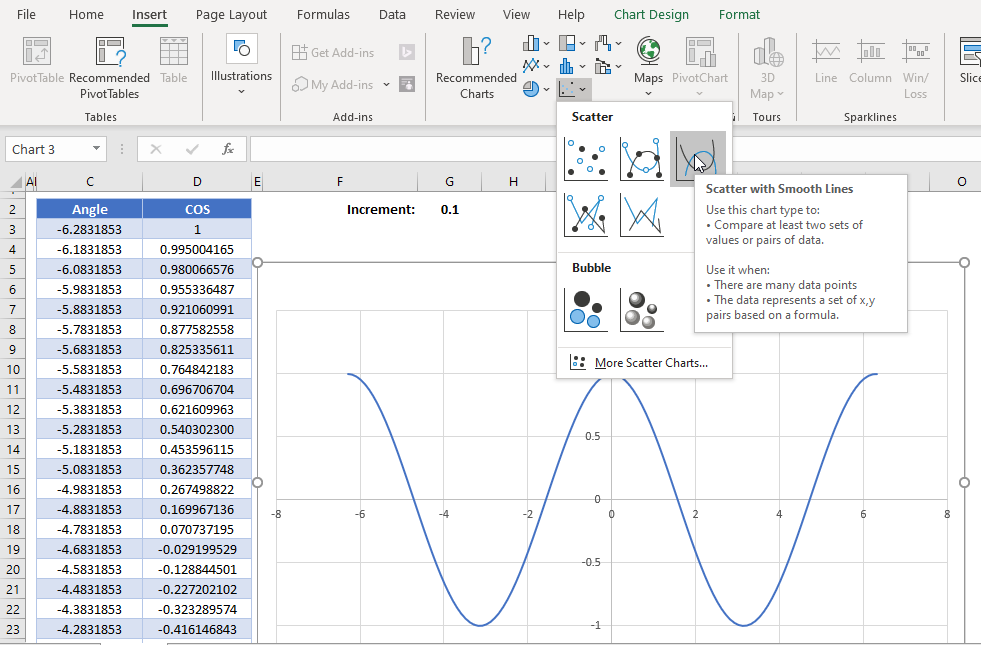### Manipulating the cosine curve

Trigonometric curves can be manipulated in a few ways – vertical shift (shifting the curve up and down on the graph), phase shift (shifting the curve left and right), amplitude modulation (how high and low the peaks and troughs are), and wavelength/period modulation (how far along the x-axis the curve is stretched or compressed).

While we won’t go in depth about each of these, here are the basic ways to manipulate each of these. Keep an eye on the formula bar in the examples to see how it changes the curve compared to the original curve.

1. Vertical shifting – Add a number after the function
`=COS(number)+verticalShift`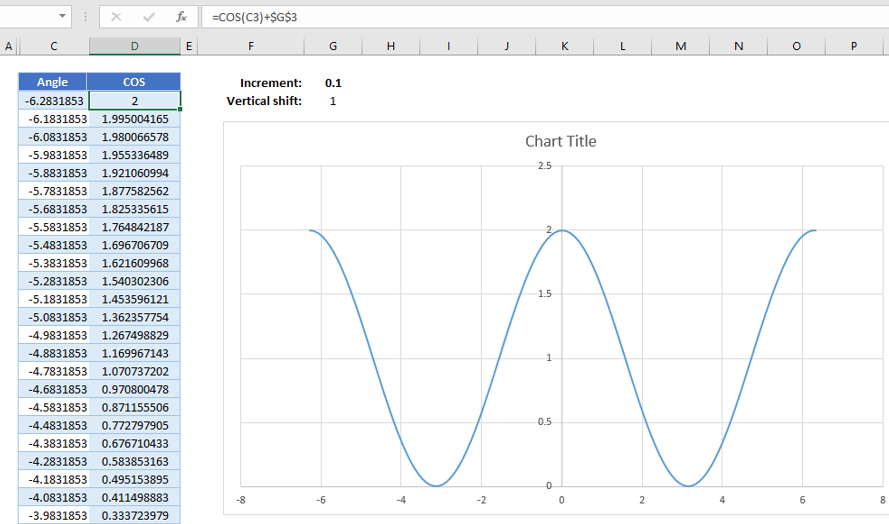2. Phase shifting – Add a number inside the argument of the function

`=COS(number+phaseShift)`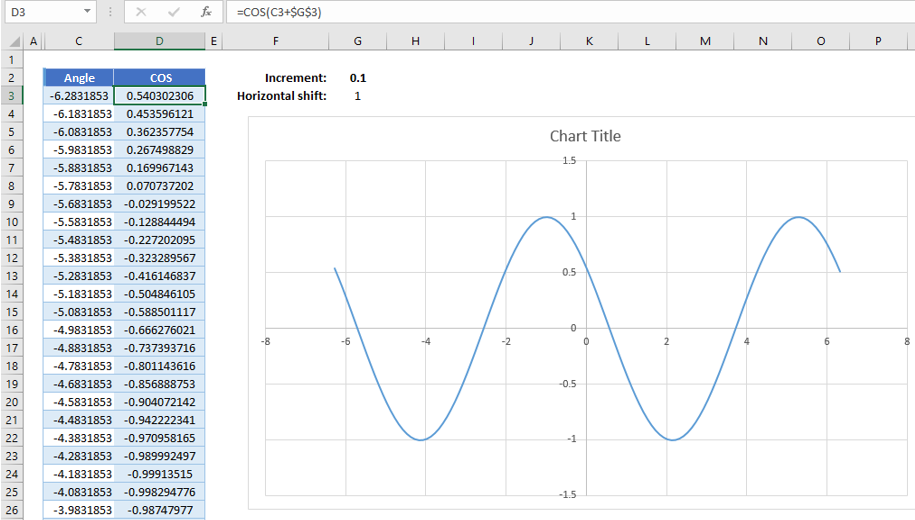3. Amplitude modulation – Multiply the function by a number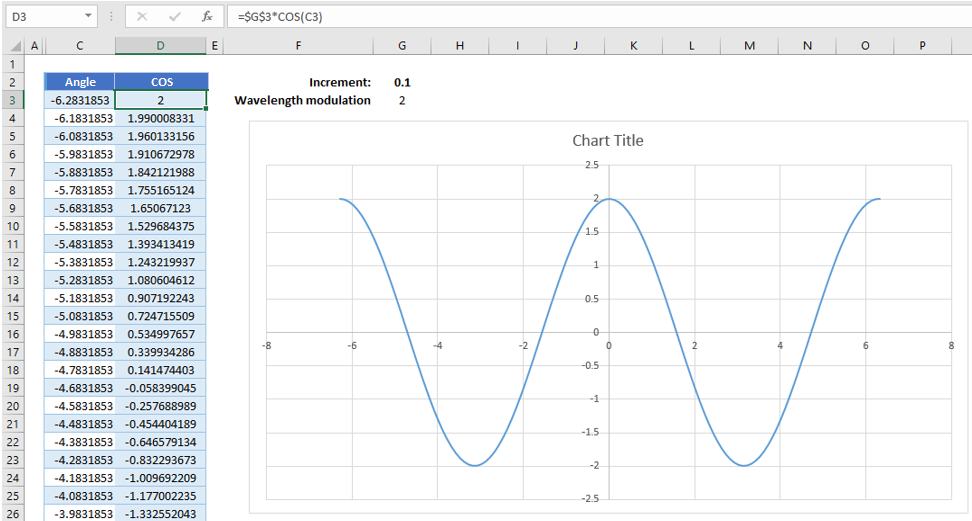4. Wavelength modulation – Multiply the argument by a number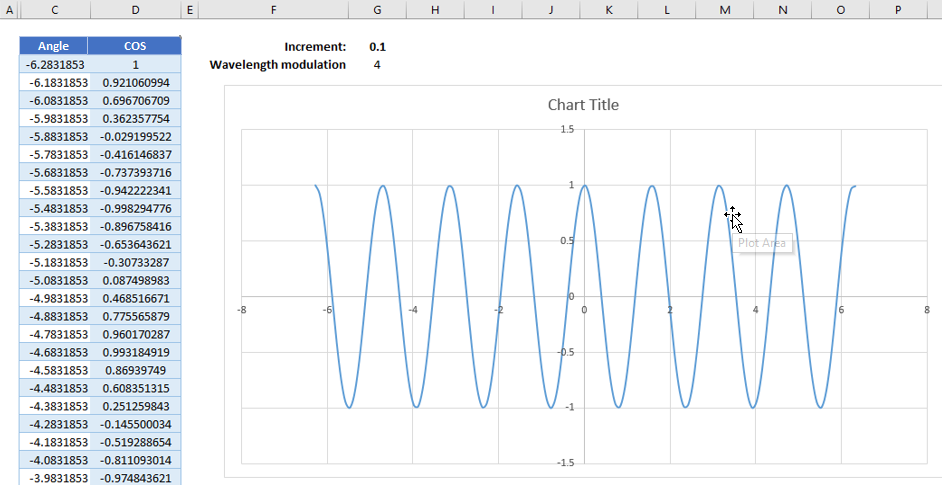## COS in Google Sheets

The COS Function works exactly the same in Google Sheets as in Excel: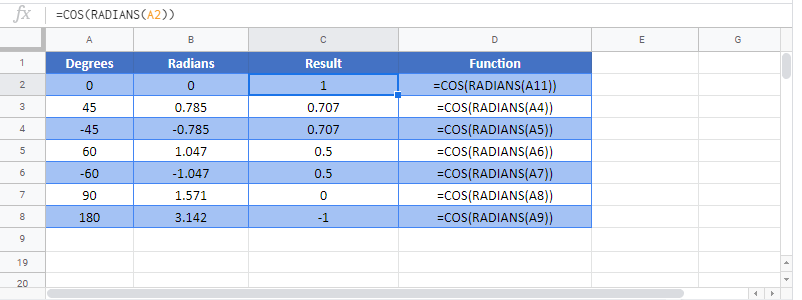### COS Examples in VBA

You can also use the COS function in VBA. Type:

```Dim val1 as double
val1 = cos(number)```

For the function arguments (number, etc.), you can either enter them directly into the function, or define variables to use as we have done here.

Common Errors in VBA

Run-time error ’13’: Type mismatch This error occurs when a non-number has been entered into the argument.

## Excel Practice Worksheet

Practice Excel functions and formulas with our 100% free practice worksheets!

• Automatically Graded Exercises
• Learn Excel, Inside Excel!